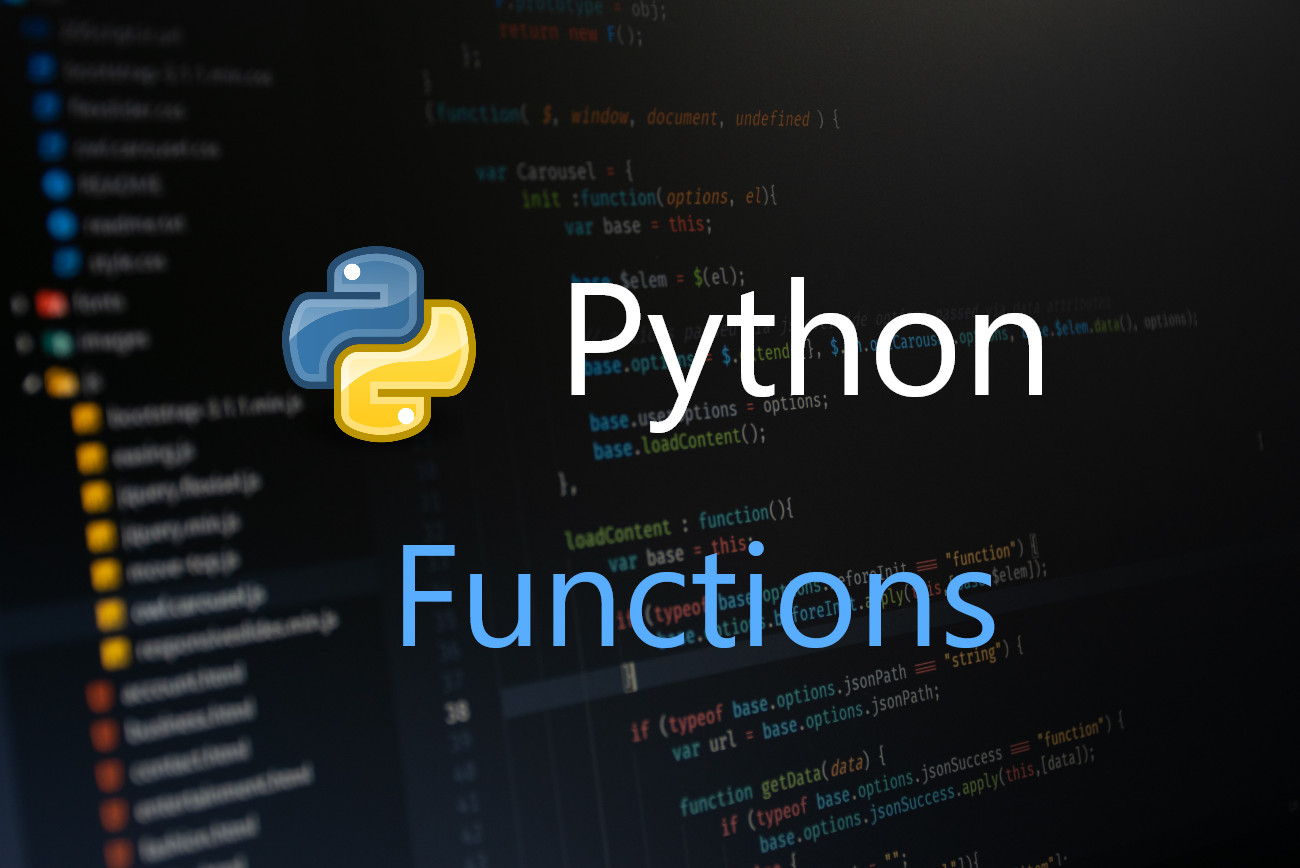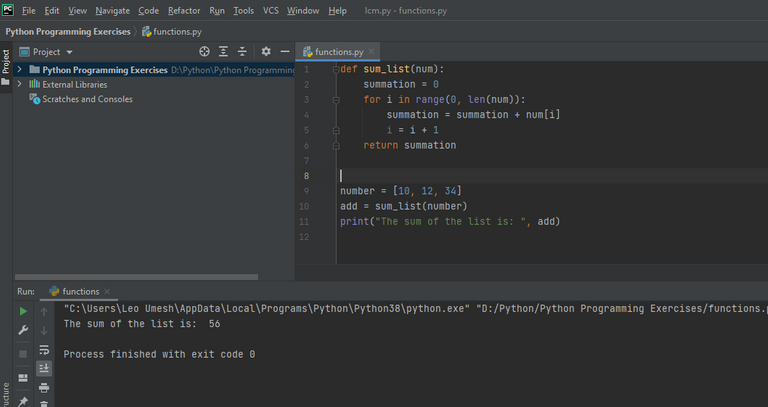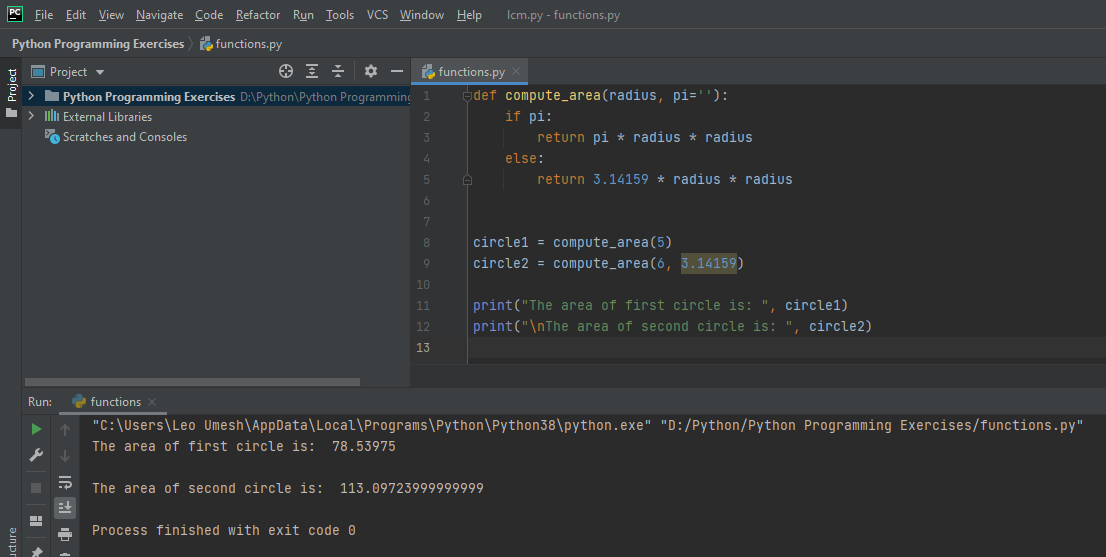# FUNCTIONS IN PYTHON PROGRAMMING (PART - 2)

in STEMGeeks4 months agoSource

For the first part of this tutorial, you can follow this link. The first part is all about introduction of a function, the simplest function structure, along types of argument values in a function. In this post we will talk about a function that returns a value and making an argument optional in Python programming.

If you want to transfer any data from the body of a function to the other part of the program then the function is said to return a value. Whenever Python encounters a return statement inside a function, it takes that value and then goes outside the function leaving the statement after return unexecuted. Let's see a simple example of return statement. We will be making a function that will return the sum of an element in a list even though python has `sum()` in-built function that can give us value within a single line of code.

``````def sum_list(num):
summation = 0
for i in range(0, len(num)):
summation = summation + num[i]
i = i + 1
return summation

number = [10, 12, 34]
print("The sum of the list is: ", add)
``````

The function will accept a list as a parameter and then it will loop through that list to find the sum of all value and at the end of the iteration, it will return a sum value of list. Whenever, you return a value from a function, you need to declare a variable to store that return value i.e. add variable in our above code. The output of this code is:In the previous post, we also talk about default values. This is one way of making argument value optional however there is another way which I am going to show you. Suppose, user doesn't want to specify any value for the argument in the program. And we want our function to continue even if user provides or doesn't provide the required number of argument as specified in the function parameter. For example: if a user wants to find the area of circle, then the value of PI is always constant i.e. 3.14159. We will create a function that will find the area of circle when user mentions the value of PI and when user doesn't mention the value of PI.

``````
if pi:
else:

circle1 = compute_area(5)
circle2 = compute_area(6, 3.14159)

print("The area of first circle is: ", circle1)
print("\nThe area of second circle is: ", circle2)
``````

You can now see the output if value of PI is provided and also when not provided. In the function definition you can see the values that should be provided should be written first as a parameter and the optional one should be always written in the last and you should specify its default value as an empty string. If the value of pi is provided then it will execute the if statement as its value is set to True and if not provided then statement inside else block is executed. Remember, here we also have return a value from the function and we are storing that returned value in the variable named as circle1 and circle2.You can try running this code online here. Let me know in the comment if you have any doubts or concerns.